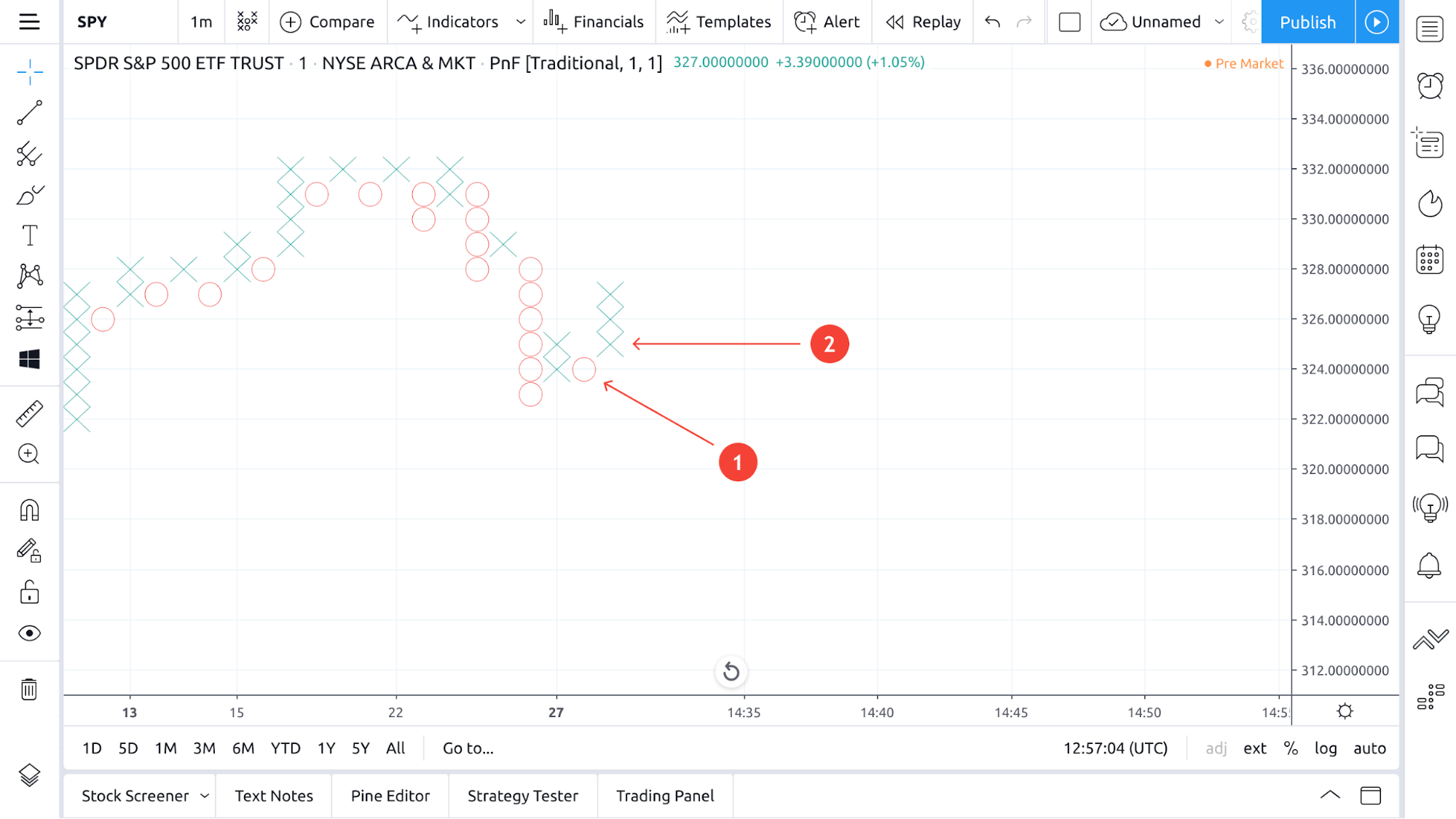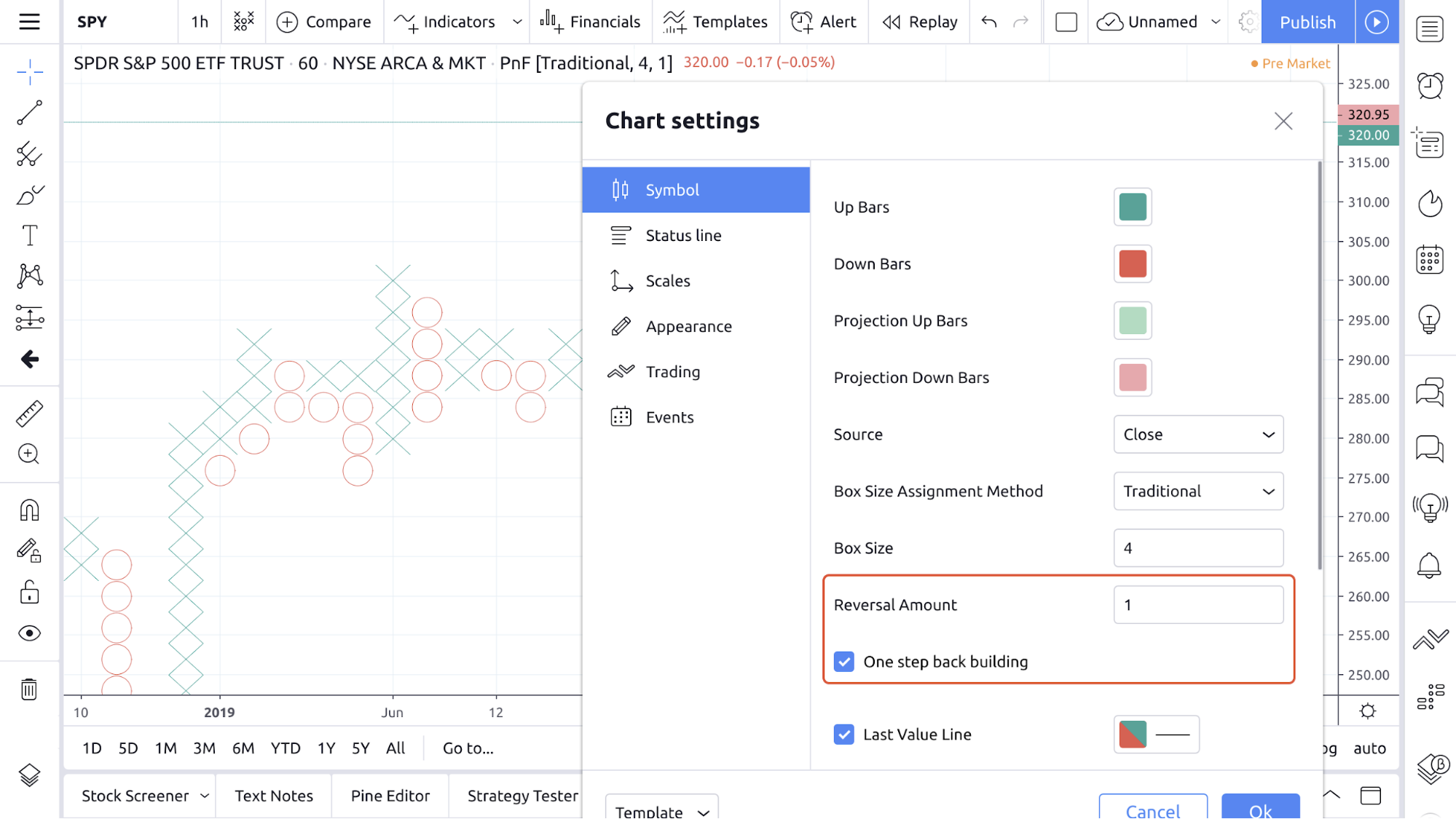# What does the One Step Back Building option mean?

One Step Back is a way to build 1-box P&F charts, which is an important component of the Wyckoff chart analysis method and helps P&F chart analysts see patterns more clearly.

In the usual construction method, figures indicating a change in price in the opposite direction are always drawn in a new column. In the picture below, these are figures O (1) and the lowest figure in column X (2).Let’s consider the One Step Back building process. If there is only one figure in the current column (O (1) in the picture), and a new figure is formed that indicates the price change in the opposite direction (in the picture this is the lowest figure in column X (2)), then this new figure is not built in a new column, rather the current one. Thus, figures X and O can be combined into one column. Keep in mind that there cannot be less than two figures.You need to set Reversal Amount = 1 and enable the One Step Back Building option to use this method on a P&F chart.Профиль Настройка профиля Счёт и оплата Ваши друзья Монеты Мои запросы в поддержку Справочный центр Личные сообщения Чат Выйти